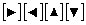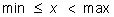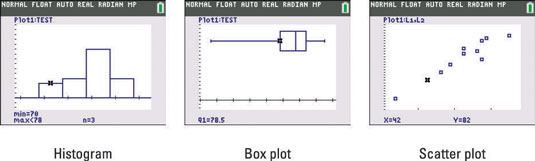##### TI-84 Plus CE Graphing Calculator For Dummies, 3rd EditionBefore tracing a statistical data plot on your TI-84 Plus , press [2nd][ZOOM] and, if necessary, highlight CoordOn in the second line of the Format menu and ExprOn in the last line. This enables you to see the name of the data set being traced and the location of the cursor. To highlight an entry, use thekeys to place the cursor on the entry and press [ENTER].

Press [TRACE] to trace a statistical data plot. In the upper-left corner of the screen, you see the Stat Plot number (P1, P2, or P3) and the name(s) of the data list(s) being traced. If you have more than one stat plot on the screen, repeatedly press the up- and down-arrow keys until the plot you want to trace appears in the upper-left corner of the screen.

Use the left- and right-arrow keys to trace the plot. What you see depends on the type of plot:

• Tracing a histogram: As you trace a histogram, the cursor moves from the top center of one bar to the top center of the next bar. At the bottom of the screen, you see the values of min, max, and n. There are n data points x such thatThis is illustrated in the first screen.• Tracing a box plot: As you trace a box plot from left to right, the values that appear at the bottom of the screen are minX (the minimum data value), Q1 (the value of the first quartile), Med (the value of the median), Q3 (the value of the third quartile, and maxX (the maximum data value). This is illustrated in the second screen.

• Tracing a modified box plot: As you trace a modified box plot from left to right, the values that appear at the bottom of the screen are minX (the minimum data value), and then you see the values of the other outliers, if any, to the left of the interval defined by 1.5(Q3 – Q1).

The next value you see at the bottom of the screen is the value of the Left Bound of the interval defined by 1.5(Q3 – Q1). Then, as with a box plot, you see the values of the first quartile, the median, and the third quartile.

After that you see the value of the right bound of the interval defined by 1.5(Q3 – Q1), the outliers to the right of this, if any, and finally, you see maxX (the maximum data value).

• Tracing a scatter plot or an xy-line plot: As you trace a scatter plot or an xy-line plot, the coordinates of the cursor location appear at the bottom of the screen. As shown in the third screen, the x-coordinate is a data value for the first data list named at the top of the screen; the y-coordinate is the corresponding data value from the second data list named at the top of the screen.